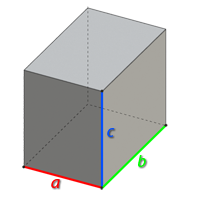# Volume of the parallelepiped

ParallelepipedCalculation formula

Formula for calculating the volume of a parallelepiped:

V = a ⋅ b ⋅ c,

where a, b, c are side lengths